内容一：

1.什么是光场？

简单的说，光场就是光线，它包含光线本身的强度、位置和方向等信息。一般采用两个相互平行的平面来表征光场，如图所示为什么要用这种双平面的方式来确定光场的分布呢？这是因为常规的相机一般都可以简化成两个互相平行的平面——镜头的光瞳面和图像传感器所在的像平面。对于常规的相机来说，每个像素记录了整个镜头所出射光线会聚在一个位置上的强度，如图所示.### 2.光场相机是怎么工作的？
光场相机由镜头、微透镜阵列和图像传感器组成，其中微透镜阵列是多个微透镜单元所组成的二维阵列。镜头的光瞳面（UV面）和图像传感器的光敏面（XY面）关于微透镜阵列（ST）成共轭关系，也就是说，镜头经过每个微透镜单元都会投影到图像传感器上形成一个小的微透镜子图像。每个微透镜子图像包含了若干个像素，此时各像素所记录的光线强度就来自于一个微透镜和镜头的一个子孔径区域之间所限制的细光束，如图。### 3.怎么实现数字对焦？
正如前面所说，获得相机内的光场分布后，就可以重新选择一个虚拟的像平面（如图，利用光场相机的数字调焦能力，只需要一次曝光就可以计算出不同像平面位置的图像，能够实现大光圈条件下的快速对焦。更进一步，利用不同深度平面的图像序列，可以完成全景深图像合成、三维深度估计等功能。

### 4.对焦示例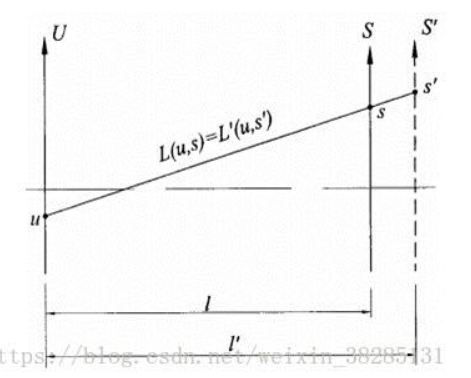S为成像面，重聚焦实际过程就是改变S的位置，使不同深度的图像清楚。S’为新的成像面，l’=alpha*l,，S’面上所成的像为US’之间光场的积分：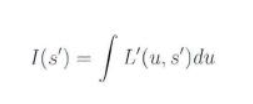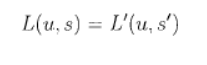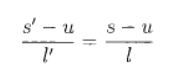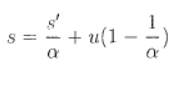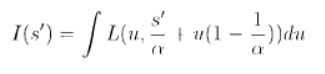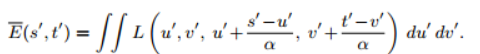void remapping
(
double * im_in_remap,//输入原始的光场图像
double * im_out_remap,//输出映射好的光场图像
double * output_image,//重聚焦图像
unsigned short width,//空间分辨率的宽
unsigned short height,//空间分辨率的高
unsigned short window_side,//角度分辨率边长或者说是微透镜直径
unsigned short stereo_diff,//大小等于微透镜半径
double         alpha//alpha就是每次改变平面的alpha值，L'=alpha*L
)
{
int                 x,y ;//空间分辨率的长和宽
unsigned int        x_1,x_2,y_1,y_2;//改变焦平面之后坐标值相邻的四个元素的左上角和右下角的像素的坐标
int                 i,j;
double              x_ind,y_ind   ;//改变焦平面之后，光线落在新的焦平面上的坐标值，一般有小数
double              x_floor,y_floor ;
double              x_1_w,x_2_w,y_1_w,y_2_w;//新的坐标值离相邻四个像素值的权重，根据距离计算
unsigned int        x_1_index,x_2_index,y_1_index,y_2_index  ;//相邻四个坐标的x和y坐标值
unsigned int        x_index_remap,y_index_remap ;//这个是Tao论文里的关于整副原始图像重聚焦的，可以忽略
double              interp_color_R,interp_color_G,interp_color_B    ;//三个通道
double              output_color_R,output_color_G,output_color_B    ;//重聚焦插值之后输出的图像
unsigned int        height_of_remap, width_of_remap, pixels_of_remap;//映射完之后原始图像高，宽，像素个数
int                 window_size                                     ;//大小为微透镜直径

window_size = window_side*window_side               ;//微透镜下面覆盖的像素值个数

height_of_remap = height*window_side                ;//子孔径图像高乘以微透镜直径
width_of_remap  = width*window_side                 ;//子孔径图像宽乘以微透镜个数
pixels_of_remap = height_of_remap*width_of_remap    ;//映射完之后的原始图像像素个数

for (x = 0; x < width; ++x)//从左到右从小到下进行遍历
for (y = 0; y < height; ++y)
{
output_color_R =0;//三个通道
output_color_G =0;
output_color_B =0;

for (i = -stereo_diff; i < stereo_diff+1; ++i)//相当于u和v，角度分辨率
for (j = -stereo_diff; j < stereo_diff+1; ++j)
{
x_ind   = i*(1-1/alpha) + x;//根据公式，新的焦平面上x的坐标  新的坐标：x+u(1-1/alpha)
y_ind   = j*(1-1/alpha) + y;//同理，y坐标   y+v(1-1/alpha)

x_floor = floor(x_ind);//向下取整
y_floor = floor(y_ind);

x_1     = index_x(x_floor  ,width );//进行边界判断
y_1     = index_y(y_floor  ,height);
x_2     = index_x(x_floor+1,width );
y_2     = index_y(y_floor+1,height);

x_1_w   = 1-(x_ind-x_floor)        ;//计算四个相邻像素插值的权重，越近权重越大
x_2_w   = 1-x_1_w                  ;
y_1_w   = 1-(y_ind-y_floor)        ;
y_2_w   = 1-y_1_w                  ;

x_1_index = i+stereo_diff + (x_1)*window_side   ;//这个是用于原始图像重聚焦的，可以忽略
y_1_index = j+stereo_diff + (y_1)*window_side   ;
x_2_index = i+stereo_diff + (x_2)*window_side   ;
y_2_index = j+stereo_diff + (y_2)*window_side   ;

interp_color_R = y_1_w*x_1_w*im_in_remap[y_1_index+x_1_index*height_of_remap+0*pixels_of_remap]+//对三个通道根据四个相邻像素进行插值
y_2_w*x_1_w*im_in_remap[y_2_index+x_1_index*height_of_remap+0*pixels_of_remap]+
y_1_w*x_2_w*im_in_remap[y_1_index+x_2_index*height_of_remap+0*pixels_of_remap]+
y_2_w*x_2_w*im_in_remap[y_2_index+x_2_index*height_of_remap+0*pixels_of_remap];
interp_color_G = y_1_w*x_1_w*im_in_remap[y_1_index+x_1_index*height_of_remap+1*pixels_of_remap]+
y_2_w*x_1_w*im_in_remap[y_2_index+x_1_index*height_of_remap+1*pixels_of_remap]+
y_1_w*x_2_w*im_in_remap[y_1_index+x_2_index*height_of_remap+1*pixels_of_remap]+
y_2_w*x_2_w*im_in_remap[y_2_index+x_2_index*height_of_remap+1*pixels_of_remap];
interp_color_B = y_1_w*x_1_w*im_in_remap[y_1_index+x_1_index*height_of_remap+2*pixels_of_remap]+
y_2_w*x_1_w*im_in_remap[y_2_index+x_1_index*height_of_remap+2*pixels_of_remap]+
y_1_w*x_2_w*im_in_remap[y_1_index+x_2_index*height_of_remap+2*pixels_of_remap]+
y_2_w*x_2_w*im_in_remap[y_2_index+x_2_index*height_of_remap+2*pixels_of_remap];

// CORRESPONDENCE ANALYSIS
x_index_remap = i+stereo_diff + (x)*window_side   ;
y_index_remap = j+stereo_diff + (y)*window_side   ;

im_out_remap[y_index_remap + x_index_remap*height_of_remap + 0*pixels_of_remap] = interp_color_R;
im_out_remap[y_index_remap + x_index_remap*height_of_remap + 1*pixels_of_remap] = interp_color_G;
im_out_remap[y_index_remap + x_index_remap*height_of_remap + 2*pixels_of_remap] = interp_color_B;

// DEFOCUS ANALYSIS
output_color_R = interp_color_R + output_color_R;//该alpha值下各个通道计算之后的单通道图像
output_color_G = interp_color_G + output_color_G;
output_color_B = interp_color_B + output_color_B;

}
output_image[y + x * height + 0 * height*width] = output_color_R/window_size;//三通道重聚焦图像
output_image[y + x * height + 1 * height*width] = output_color_G/window_size;
output_image[y + x * height + 2 * height*width] = output_color_B/window_size;

}
}

2.Tao在论文里面进行了256层重聚焦，alpha范围为（0.2,2），因此每一次改变焦平面，alpha值增加（2-0.2）/256
3.他输入的中心点坐标image_cords是[m,n,2]，2代表横坐标，1是纵坐标，这个需要注意。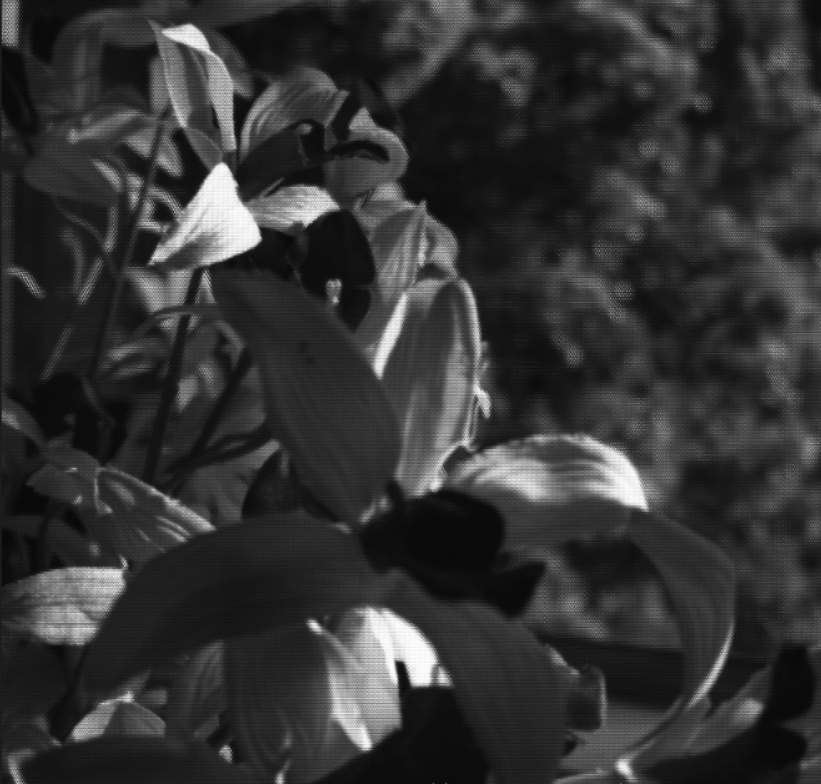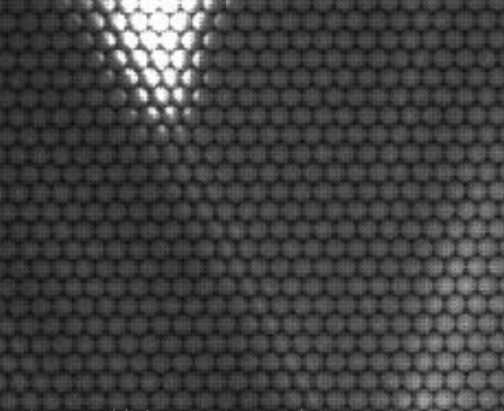alpha=0.2时：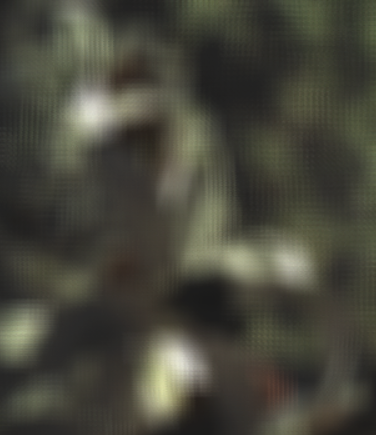alpha=0.2+（1.8/256）*78时：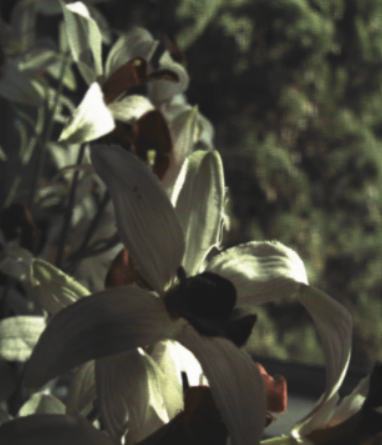alpha=2时：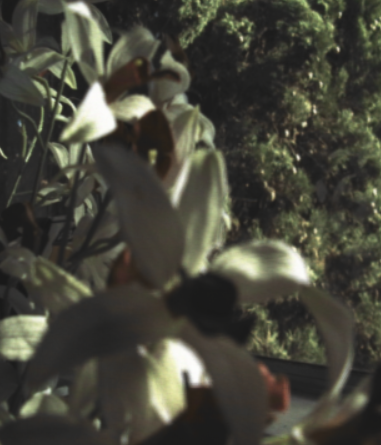1.光场成像技术研究-周志良
2.Light Field Photography with a Hand-held Plenoptic Camera–Ren-Ng
3.Depth from Combining Defocus and Correspondence Using Light-Field Cameras-W.Tao
4.https://www.cnblogs.com/riddick/p/6731130.html